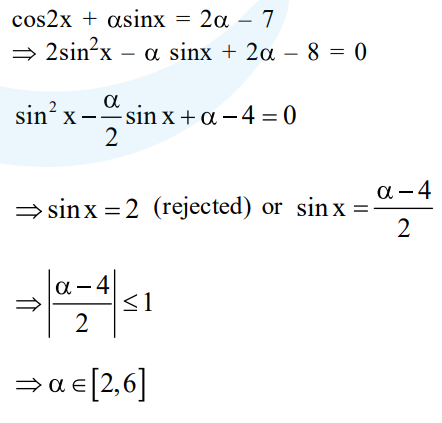# Let S be the set of all`
Question:

Let $\mathrm{S}$ be the set of all $\alpha \in R$ such that the equation, $\cos 2 x+\alpha \sin x=2 \alpha-7$ has a solution. Then $S$ is equal to :

1. $[2,6]$

2. $[3,7]$

3. $R$

4. $[1,4]$

Correct Option: 1

Solution: